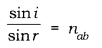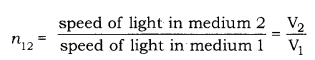# (a) State the laws of refraction of light

(a) State the laws of refraction of light. Give an expression to relate the absolute refractive index of a medium with speed of light in vacuum.
(b) The refractive indices of water and glass with respect to air are 4/3 and 3/2 respectively. If the speed of light in glass is 2 x 108 ms4, find the speed of light in

1. air
2. water.

(a) Laws of refraction: There are two laws of refraction.

First law of refraction: The ratio of the sine of the angle of incidence to the sine of the angle of refraction is constant. This is known as Snell’s law. Mathematically, it can be given as follows:Here, nab is the relative refractive index of medium a with respect to medium b.

Second law of refraction: The incident ray, the refracted ray, and the normal to the interface of two media at the point of incidence - all lie in the same plane.
If the light ray goes from medium 1 to 2, then the refractive index of medium 1 with respect to medium 2 is,where, V1 and V2 are the speeds of light in medium 1 and 2 respectively.

(b) Given: Refractive index of water, nw =4/3 and Refractive index of glass, ng =3/2.
Speed of light in glass, vg = 2 × 108 m/s